### Home > CALC > Chapter 7 > Lesson 7.3.5 > Problem7-156

7-156.
1. Stingray populations grow based on the differential equation below, where P is the population (in thousands) and t is time in years. Use the graph of the slope field at right to answer the following questions. Homework Help ✎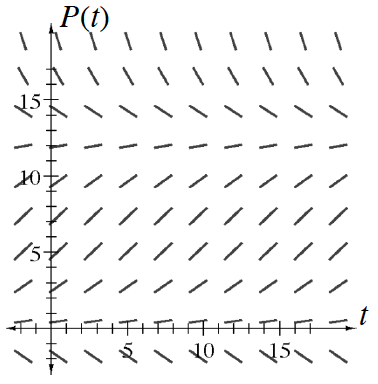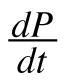= 0.026P(12.5 − P)

1. If there are 3,000 stingrays at time t = 0, sketch a curve representing their population of stingrays.

2. What if the original population at t = 0 is 10,000 stingrays? Draw that population curve and decide if its growth is the same or different as that in part (a).

3. What if the original population at t = 0 is 17,000 stingrays? Draw that population curve and decide if its growth is the same or different as those in parts (a) and (b).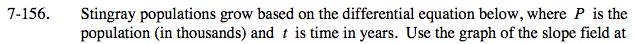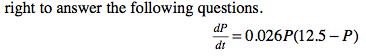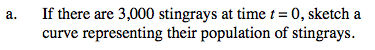The starting point of the curve is (0, 3000).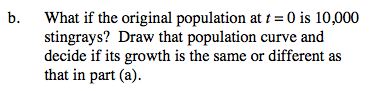The starting point of the curve is (0, 10,000).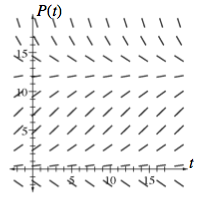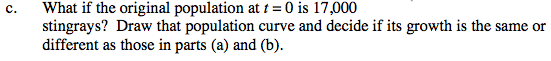Based on parts (a) and (b), you should have noticed that both curves approached the same value over time.
The starting point of this curve is (0, 17,000). Do you think it will approach the same threshold?